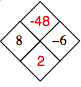### Home > AC > Chapter Ch3 > Lesson 3.2.4 > Problem3-100

3-100.

Copy and complete each of the Diamond Problems below. The pattern used in the Diamond Problems is shown below. Homework Help ✎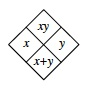1.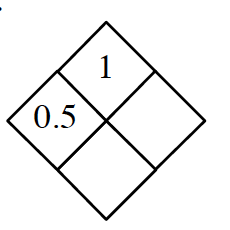Divide 1 by 0.5 to find $y$.

Add $x$ and $y$ together to get the bottom of the diamond.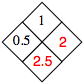1.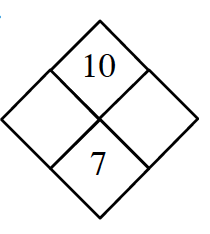What are the factors of 10?

Which of those add to 7?

1.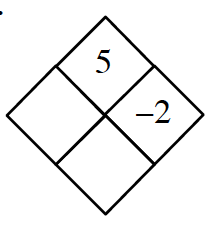Follow the steps in part (a).

1.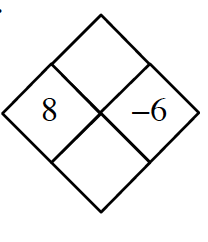Use the pattern above to find $xy$ and $x+y$.